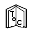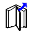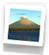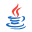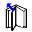## X3D Example Archives: X3D for Advanced Modeling, Matlab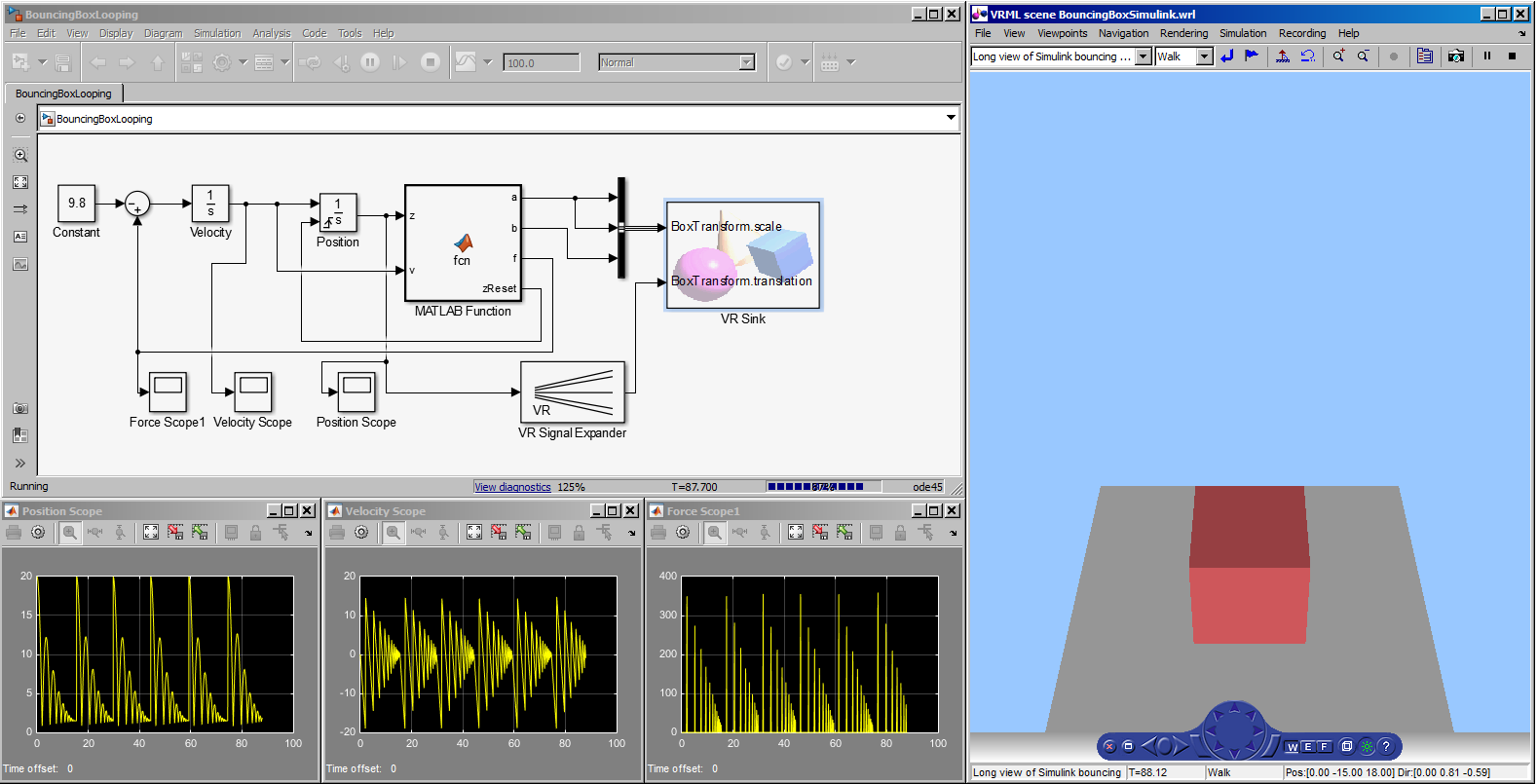The Matlab toolkit from Mathworks includes numerous mathematical and scientific packages. Simulink can be used for animation visualization using VRML models, which are easily created from X3D. X3D models can be converted to VRML for use with Simulink and other Matlab tools. Web3D 2015 conference resources: Simulink demo, poster paper, Fast Forward slides (.pdf) and video.
2 X3D Models       X3D Model DescriptionsBouncing Box Simulink Bounce a flexible Box up and down by adapting an example from Mathworks Matlab using Simulink for animation.Phased Array Beam Example Conversion Matlab figure to x3d using conversion method number 2.